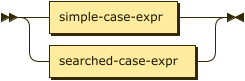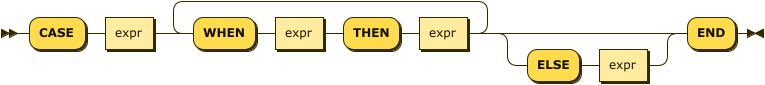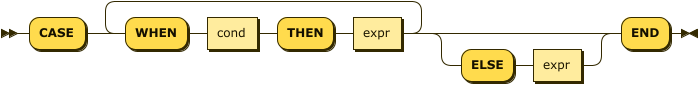A newer version of this documentation is available.

# Conditional Operators

• reference
+

Case expressions evaluate conditional logic in an expression.

case-expr:

`simple-case-expression | searched-case-expression`## Simple Case Expressions

simple-case-expr:

```CASE expression  ( WHEN expression THEN expression)
[ ( WHEN expression THEN expression) ]*
[  ELSE expression ]  END```Simple case expressions allow for conditional matching within an expression. The evaluation process is as follows:

• The first WHEN expression is evaluated. If it is equal to the search expression, the result of this expression is the THEN expression.

• If it is not equal, subsequent WHEN clauses are evaluated in the same manner.

• If none of the WHEN expressions are equal to the search expression, then the result of the CASE expression is the ELSE expression.

• If no ELSE expression was provided, the result is NULL.

## Searched Case Expressions

searched-case-expression:

```CASE  ( WHEN  condition THEN expression)
[( WHEN  condition THEN expression ) ]*
[ ELSE  expression ] END```Searched case expressions allow for conditional logic within an expression. The evaluation process is as follows:

• The first WHEN expression is evaluated.

• If TRUE, the result of this expression is the THEN expression.

• If not TRUE, subsequent WHEN clauses are evaluated in the same manner.

• If none of the WHEN clauses evaluate to TRUE, then the result of the expression is the ELSE expression.

• If no ELSE expression was provided, the result is NULL.

### Example

The following example uses a CASE clause to handle documents that do not have a ship date. This scans all orders. If an order has a shipped-on date, it is provided in the result set. If an order does not have a shipped-on date, default text appears.

Query
``````SELECT
CASE WHEN `shipped-on`
IS NOT NULL THEN `shipped-on`
ELSE "not-shipped-yet"
END
AS shipped
FROM orders``````
Result
``````{ "shipped": "2013/01/02" },
{ "shipped": "2013/01/12" },
{ "shipped": "not-shipped-yet" },``````
Output